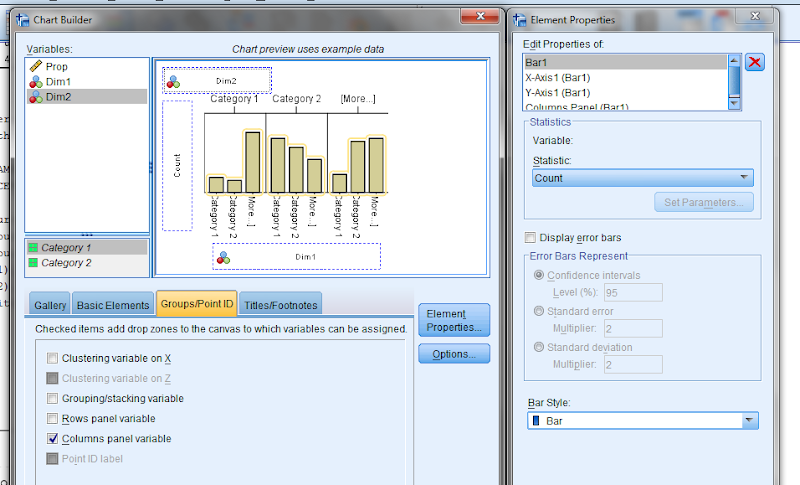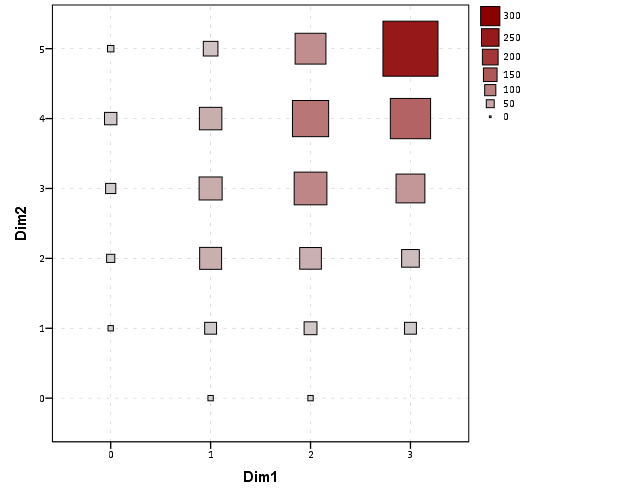# Fluctuation diagrams in SPSS

The other day on my spineplot post Jon Peck made a comment about how he liked the structure charts in this CV thread. Here I will show how to make them in SPSS (FYI the linked thread has an example of how to make them in R using ggplot2 if you are interested).

Unfortunately I accidently called them a structure plot in the original CV thread, when they are actually called fluctuation diagrams (see Pilhofer et al. (2012) and Wickham & Hofmann (2011) for citations). They are basically just binned scatterplots for categorical data, and the size of a point is mapped to the number of observations that fall within that bin. Below is the example (in ggplot2) taken from the CV thread.So, to make these in SPSS you first need some categorical data, you can follow along with any two categorical variables (or at the end of the post I have the complete syntax with some fake categorical data). First, it is easier to start with some boilerplate code generated through the GUI. If you have any data set open that has categorical data in it (unaggregated) you can simply open up the chart builder dialog, choose a barplot, place the category you want on the x axis, then place the category you want on the Y axis as a column panel for a paneled bar chart.The reason you make this chart is that the GUI interprets the default behavior of this bar chart is to aggregate the frequencies. You make the colum panel just so the GUI will write out the data definitions for you. If you pasted the chart builder syntax then the GPL code will look like below.

``````
*Fluctuation plots - first make column paneled bar chart.
GGRAPH
/GRAPHDATASET NAME="graphdataset" VARIABLES=Dim1[LEVEL=NOMINAL] COUNT()
[name="COUNT"] Dim2[LEVEL=NOMINAL] MISSING=LISTWISE REPORTMISSING=NO
/GRAPHSPEC SOURCE=INLINE.
BEGIN GPL
SOURCE: s=userSource(id("graphdataset"))
DATA: Dim1=col(source(s), name("Dim1"), unit.category())
DATA: COUNT=col(source(s), name("COUNT"))
DATA: Dim2=col(source(s), name("Dim2"), unit.category())
GUIDE: axis(dim(1), label("Dim1"))
GUIDE: axis(dim(2), label("Count"))
GUIDE: axis(dim(3), label("Dim2"), opposite())
SCALE: cat(dim(1))
SCALE: linear(dim(2), include(0))
SCALE: cat(dim(3))
ELEMENT: interval(position(Dim1*COUNT*Dim2), shape.interior(shape.square))
END GPL.
``````

With this boilerplate code though we can edit to make the chart we want. Here I outline some of those steps. Only editing the `ELEMENT` portion, the steps below are;

• Edit the `ELEMENT` statement to be a `point` instead of `interval`.
• Delete `COUNT` within the position statement (within the `ELEMENT`).
• Change `shape.interior` to `shape`.
• Add in `,size(COUNT)` after `shape(shape.square)`.
• Add in `,color.interior(COUNT)` after `size(COUNT)`.

Those are all of the necessary statements to produce the fluctuation chart. The next two are to make the chart look nicer though.

• Add in aesthetic mappings for scale statements (both the color and the size).
• Change the guide statements to have the correct labels (and delete the dim(3) GUIDE).

The GPL code call then looks like this (with example aesthetic mappings) and below that is the chart it produces.

``````
*In the end fluctuation plot.
GGRAPH
/GRAPHDATASET NAME="graphdataset" VARIABLES=Dim1 Dim2 COUNT()[name="COUNT"]
/GRAPHSPEC SOURCE=INLINE.
BEGIN GPL
SOURCE: s=userSource(id("graphdataset"))
DATA: Dim1=col(source(s), name("Dim1"), unit.category())
DATA: Dim2=col(source(s), name("Dim2"), unit.category())
DATA: COUNT=col(source(s), name("COUNT"))
GUIDE: axis(dim(1), label("Dim1"))
GUIDE: axis(dim(2), label("Dim2"))
SCALE: pow(aesthetic(aesthetic.size), aestheticMinimum(size."8px"), aestheticMaximum(size."45px"))
SCALE: linear(aesthetic(aesthetic.color.interior), aestheticMinimum(color.lightgrey), aestheticMaximum(color.darkred))
ELEMENT: point(position(Dim1*Dim2), shape(shape.square), color.interior(COUNT), size(COUNT))
END GPL.
``````Aesthetically, besides the usual niceties the only thing to note is that the size of the squares typically needs to be changed to fill up the space (you would have to be lucky to have an exact mapping between area and the categorical count to work out). I presume squares are preferred because area assessments with squares tend to be more accurate than circles, but that is just my guess (you could use any shape you wanted). I use a power scale for size aesthetic, as the area for a square increases by the size of the side squared (and people interpret the areas in the plot, not the size of the side of the square). SPSS’s default exponent for a power scale is 0.5, which is the square root so exactly what we want. You just need to supply a reasonable start and end size for the squares to let them fill up the space depending on your counts. Unfortunately, SPSS does not make a correctly scaled legend in size, but the color aesthetic is correct (I leave it in only to show that it is incorrect, if for publication I would like suppress the different sizes and only show the color gradient). (Actually, my V20 continues to not respect shape aesthetics that aren’t mapped – and this is produced via post-hoc editing of the shape – owell).

Here I show two redundant continuous aesthetic scales (size and color). SPSS’s behavior is to make the legend discrete instead of continuous. In Wilkinson’s Grammar of Graphics he states that he prefers discrete scales (even for continous aesthetics) to aid lookup.

``````
***********************************************************.
*Making random categorical data.
set seed 14.
input program.
loop #i = 1 to 1000.
compute Prop = RV.UNIFORM(.5,1).
end case.
end loop.
end file.
end input program.
dataset name cats.
exe.

compute Dim1 = RV.BINOMIAL(3,PROP).
compute Dim2 = RV.BINOMIAL(5,PROP).

*Fluctuation plots - first make column paneled bar chart.
GGRAPH
/GRAPHDATASET NAME="graphdataset" VARIABLES=Dim1[LEVEL=NOMINAL] COUNT()
[name="COUNT"] Dim2[LEVEL=NOMINAL] MISSING=LISTWISE REPORTMISSING=NO
/GRAPHSPEC SOURCE=INLINE.
BEGIN GPL
SOURCE: s=userSource(id("graphdataset"))
DATA: Dim1=col(source(s), name("Dim1"), unit.category())
DATA: COUNT=col(source(s), name("COUNT"))
DATA: Dim2=col(source(s), name("Dim2"), unit.category())
GUIDE: axis(dim(1), label("Dim1"))
GUIDE: axis(dim(2), label("Count"))
GUIDE: axis(dim(3), label("Dim2"), opposite())
SCALE: cat(dim(1))
SCALE: linear(dim(2), include(0))
SCALE: cat(dim(3))
ELEMENT: interval(position(Dim1*COUNT*Dim2), shape.interior(shape.square))
END GPL.

*Then edit 1) element to point.
*2) delete COUNT within position statement
*3) shape.interior -> shape
*6) add in aesthetic mappings for scale statements
*7) change guide statements - then you are done.

*In the end fluctuation plot.
GGRAPH
/GRAPHDATASET NAME="graphdataset" VARIABLES=Dim1 Dim2 COUNT()[name="COUNT"]
/GRAPHSPEC SOURCE=INLINE.
BEGIN GPL
SOURCE: s=userSource(id("graphdataset"))
DATA: Dim1=col(source(s), name("Dim1"), unit.category())
DATA: Dim2=col(source(s), name("Dim2"), unit.category())
DATA: COUNT=col(source(s), name("COUNT"))
GUIDE: axis(dim(1), label("Dim1"))
GUIDE: axis(dim(2), label("Dim2"))
SCALE: pow(aesthetic(aesthetic.size), aestheticMinimum(size."8px"), aestheticMaximum(size."45px"))
SCALE: linear(aesthetic(aesthetic.color.interior), aestheticMinimum(color.lightgrey), aestheticMaximum(color.darkred))
ELEMENT: point(position(Dim1*Dim2), shape(shape.square), color.interior(COUNT), size(COUNT))
END GPL.

*Alternative ways to map sizes in the plot.
*SCALE: linear(aesthetic(aesthetic.size), aestheticMinimum(size."5%"), aestheticMaximum(size."30%")).
*SCALE: linear(aesthetic(aesthetic.size), aestheticMinimum(size."6px"), aestheticMaximum(size."18px")).

*Alternative jittered scatterplot - need to remove the agg variable COUNT.
*Replace point with point.jitter.
GGRAPH
/GRAPHDATASET NAME="graphdataset" VARIABLES=Dim1 Dim2
/GRAPHSPEC SOURCE=INLINE.
BEGIN GPL
SOURCE: s=userSource(id("graphdataset"))
DATA: Dim1=col(source(s), name("Dim1"), unit.category())
DATA: Dim2=col(source(s), name("Dim2"), unit.category())
GUIDE: axis(dim(1), label("Dim1"))
GUIDE: axis(dim(2), label("Dim2"))
ELEMENT: point.jitter(position(Dim1*Dim2))
END GPL.
***********************************************************.
``````